# All You Need to Know about Gradient Boosting Algorithm − Part 1: Regression

Machine LearningModelingposted by ODSC Community August 17, 2022

Gradient boosting is one of the most popular machine learning algorithms for tabular datasets. It is powerful enough to find any...Gradient boosting is one of the most popular machine learning algorithms for tabular datasets. It is powerful enough to find any nonlinear relationship between your model target and features and has great usability that can deal with missing values, outliers, and high cardinality categorical values on your features without any special treatment. While you can build barebone gradient boosting trees using some popular libraries such as XGBoost or LightGBM without knowing any details of the algorithm, you still want to know how it works when you start tuning hyper-parameters, customizing the loss functions, etc., to get better quality on your model.

This article aims to provide you with all the details about the algorithm, specifically its regression algorithm, including its math and Python code from scratch. If you are more interested in the classification algorithm, please look at Part 2.

## Algorithm with an Example

Gradient boosting is one of the variants of ensemble methods where you create multiple weak models and combine them to get better performance as a whole.

In this section, we are building gradient boosting regression trees step by step using the below sample which has a nonlinear relationship between `x` and `y` to intuitively understand how it works (all the pictures below are created by the author).

Sample for a regression problem

The first step is making a very naive prediction on the target `y`. We make the initial prediction `F₀` as an overall average of `y`:

Initial prediction: F0 = mean(y)

You might feel using the mean for the prediction is silly, but don’t worry. We will improve our prediction as we add more weak models to it.

To improve our prediction, we will focus on the residuals (i.e. prediction errors) from the first step because that is what we want to minimize to get a better prediction. The residuals `r₁` are shown as the vertical blue lines in the figure below.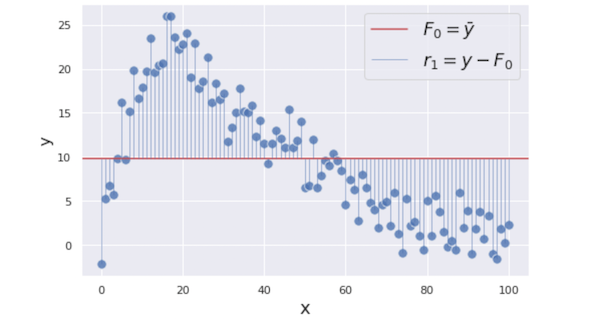To minimize these residuals, we are building a regression tree model with `x` as its feature and the residuals `r₁ = y − mean(y)` as its target. The reasoning behind that is if we can find some patterns between `x` and `r₁` by building the additional weak model, we can reduce the residuals by utilizing it.

To simplify the demonstration, we are building very simple trees each of that only has one split and two terminal nodes which is called “stump”. Please note that gradient boosting trees usually have a little deeper trees such as ones with 8 to 32 terminal nodes.

Here we are creating the first tree predicting the residuals with two different values `γ₁ = {6.0, −5.9}`(we are using `γ`(gamma) to denotes the prediction).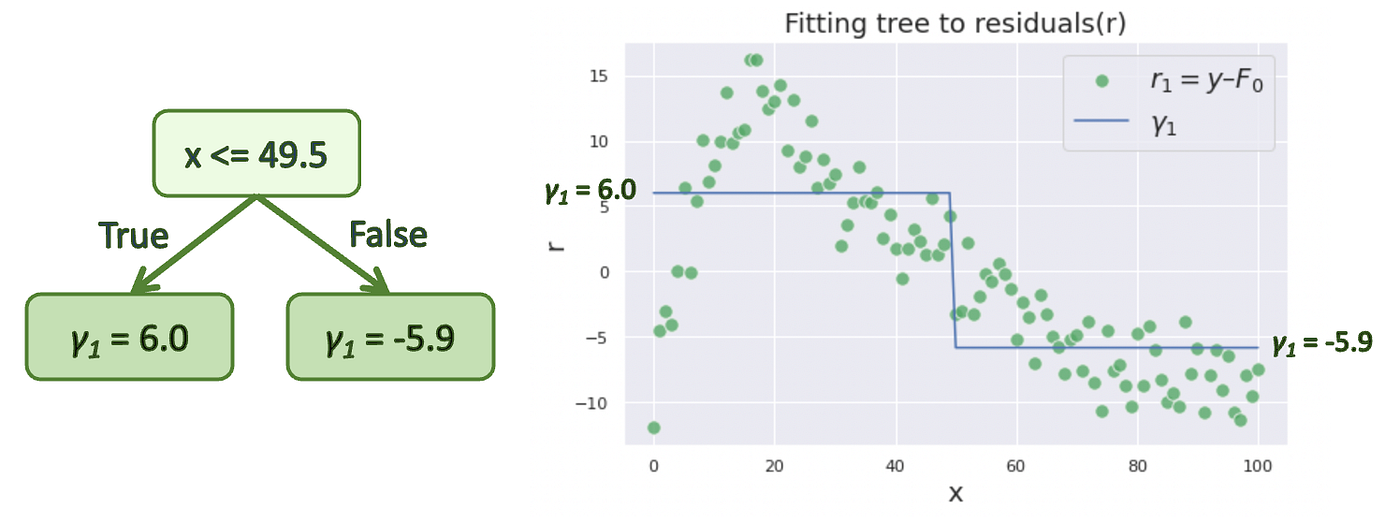This prediction `γ₁` is added to our initial prediction `F₀` to reduce the residuals. In fact, the gradient boosting algorithm does not simply add `γ` to `F` as it makes the model overfit to the training data. Instead, `γ` is scaled down by learning rate `ν` which ranges between 0 and 1, and then added to `F`.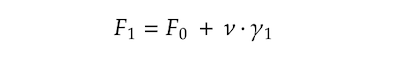In this example, we use a relatively big learning rate `ν = 0.9` to make the optimization process easier to understand, but it is usually supposed to be a much smaller value such as 0.1.

After the update, our combined prediction `F₁` becomes:Now, the updated residuals `r₂` looks like this: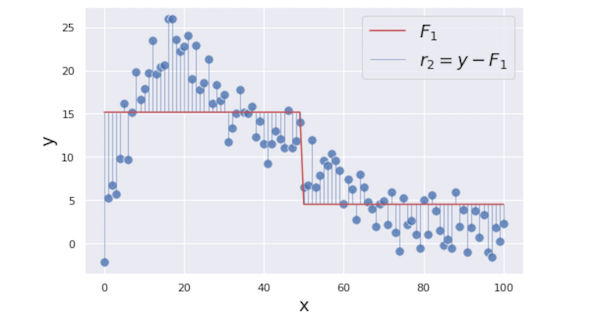In the next step, we are creating a regression tree again using the same `x` as the feature and the updated residuals `r₂` as its target. Here is the created tree: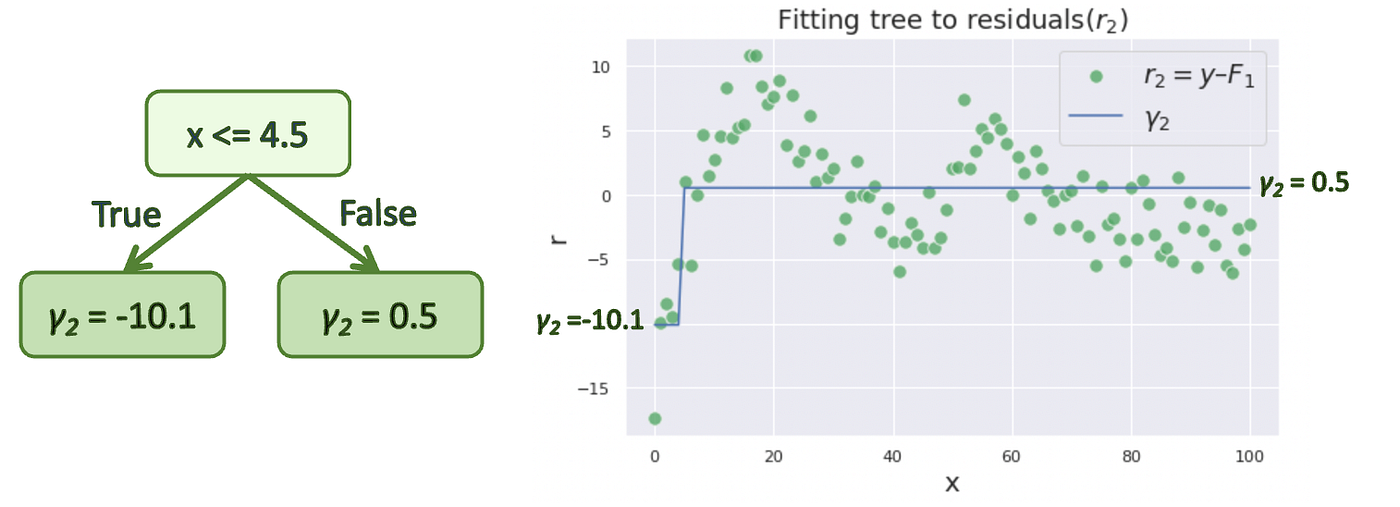Then, we are updating our previous combined prediction `F₁` with the new tree prediction `γ₂`.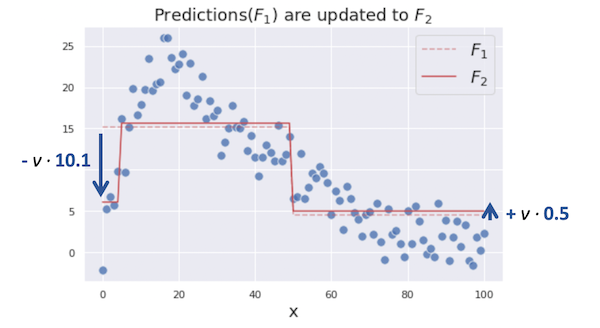We iterate these steps until the model prediction stops improving. The figures below show the optimization process from 0 to 6 iterations.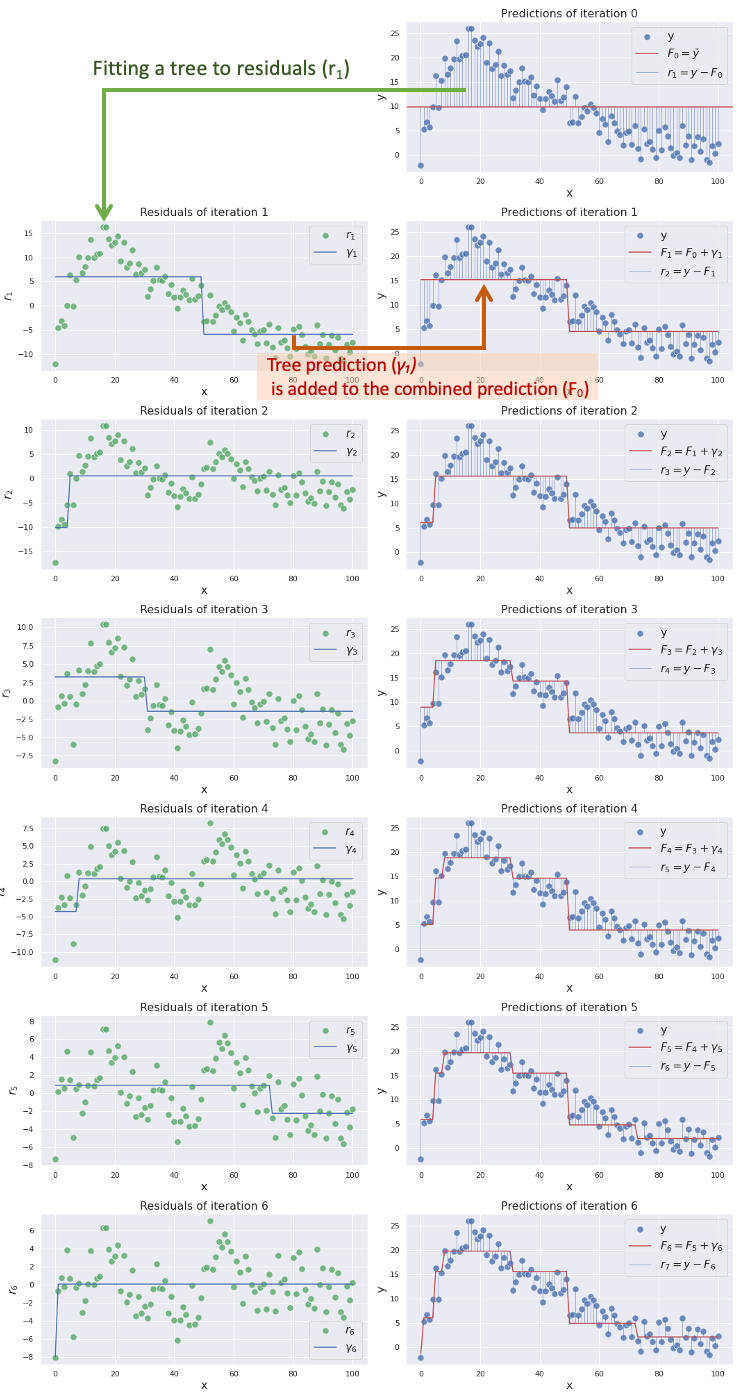You can see the combined prediction `F𝑚` is getting more closer to our target `y` as we add more trees into the combined modelThis is how gradient boosting works to predict complex targets by combining multiple weak models.

## Math

In this section, we are diving into the math details of the algorithm. Here is the whole algorithm in math formulas.

Source: adapted from Wikipedia and Friedman’s paper

Let’s demystify this line by line.

### Step 1The first step is creating an initial constant value prediction `F₀``L` is the loss function and it is squared loss in our regression case.`argmin`means we are searching for the value `γ`that minimizes`ΣL(yᵢ,γ)`. Let’s compute the value `γ` by using our actual loss function. To find`γ`that minimizes `ΣL`, we are taking a derivative of `ΣL` with respect to `γ`.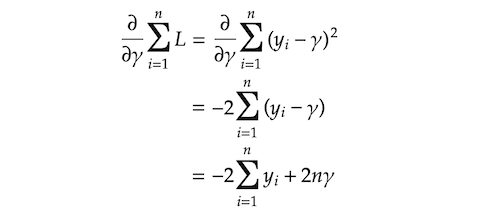And we are finding `γ`that makes `∂ΣL/∂γ` equal to 0.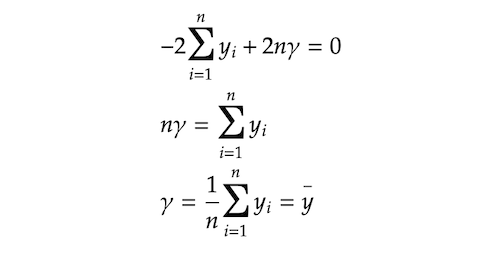It turned out that the value `γ` that minimizes `ΣL` is the mean of `y`. This is why we used `y` mean for our initial prediction `F₀` in the last section.### Step2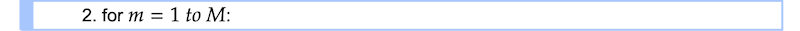The whole step2 processes from 2–1 to 2–4 are iterated `M` times. `M` denotes the number of trees we are creating and the small `m` represents the index of each tree.

### Step 2–1We are calculating residuals `rᵢ𝑚` by taking a derivative of the loss function with respect to the previous prediction `F𝑚-₁` and multiplying it by −1. As you can see in the subscript index, `rᵢ𝑚` is computed for each single sample `i`. Some of you might be wondering why we are calling this `rᵢ𝑚` residuals. This value is actually negative gradient that gives us guidance on the directions (+/−) and the magnitude in which the loss function can be minimized. You will see why we are calling it residuals shortly. By the way, this technique where you use a gradient to minimize the loss on your model is very similar to gradient descent technique which is typically used to optimize neural networks. (In fact, they are slightly different from each other. If you are interested, please look at this article detailing that topic.)

Let’s compute the residuals here. `F𝑚-₁` in the equation means the prediction from the previous step. In this first iteration, it is `F₀`. We are solving the equation for residuals `rᵢ𝑚`.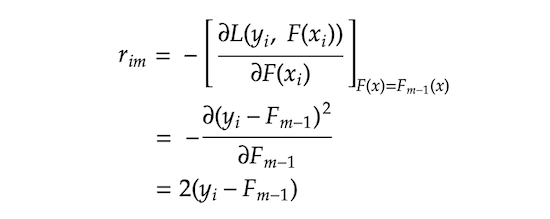We can take 2 out of it as it is just a constant. That leaves us `rᵢ𝑚 = yᵢ − F𝑚-₁`. You might now see why we call it residuals. This also gives us interesting insight that the negative gradient that provides us the direction and the magnitude to which the loss is minimized is actually just residuals.

### Step 2–2`j` represents a terminal node (i.e. leave) in the tree, `m` denotes the tree index, and capital `J` means the total number of leaves.

### Step 2–3We are searching for `γⱼ𝑚` that minimizes the loss function on each terminal node `j``Σxᵢ∈Rⱼ𝑚 L` means we are aggregating the loss on all the sample `xᵢ`s that belong to the terminal node `Rⱼ𝑚`. Let’s plugin the loss function into the equation.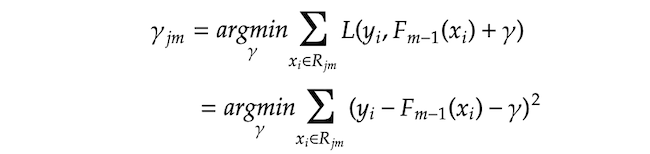Then, we are finding `γⱼ𝑚` that makes the derivative of Σ(*) equals zero.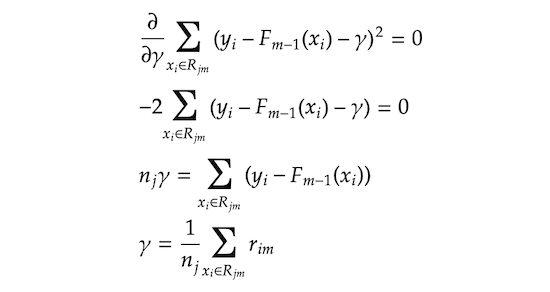Please note that `nⱼ` means the number of samples in the terminal node `j`. This means the optimal `γⱼ𝑚` that minimizes the loss function is the average of the residuals `rᵢ𝑚` in the terminal node `Rⱼ𝑚`. In other words, `γⱼ𝑚` is the regular prediction values of regression trees that are the average of the target values (in our case, residuals) in each terminal node.

### Step 2–4In the final step, we are updating the prediction of the combined model `F𝑚``γⱼ𝑚1(x ∈ Rⱼ𝑚)` means that we pick the value `γⱼm` if a given `x` falls in a terminal node `Rⱼ𝑚`. As all the terminal nodes are exclusive, any given single `x` falls into only a single terminal node and corresponding `γⱼ𝑚` is added to the previous prediction `F𝑚-₁` and it makes updated prediction `F𝑚`.

As mentioned in the previous section, `ν` is learning rate ranging between 0 and 1 which controls the degree of contribution of the additional tree prediction `γ` to the combined prediction `F𝑚`. A smaller learning rate reduces the effect of the additional tree prediction, but it basically also reduces the chance of the model overfitting to the training data.

Now we have gone through the whole steps. To get the best model performance, we want to iterate step 2 `M` times, which means adding `M` trees to the combined model. In reality, you might often want to add more than 100 trees to get the best model performance.

Some of you might feel that all those maths are unnecessarily complex as the previous section showed the basic idea in a much simpler way without all those complications. The reason behind it is that gradient boosting is designed to be able to deal with any loss functions as long as it is differentiable and the maths we reviewed is a generalized form of gradient boosting algorithm with that flexibility. That makes the formula a little complex, but it is the beauty of the algorithm as it has huge flexibility and convenience to work on a variety of types of problems. For example, if your problem requires absolute loss instead of squared loss, you can just replace the loss function and the whole algorithm works as it is as defined above. In fact, popular gradient boosting implementations such as XGBoost or LightGBM have a wide variety of loss functions, so you can choose whatever loss functions that suit your problem (see the various loss functions available in XGBoost or LightGBM).

## Code

In this section, we are translating the maths we just reviewed into a viable python code to help us understand the algorithm further. The code is mostly derived from Matt Bowers’ implementation, so all credit goes to his work. We are using `DecisionTreeRegressor` from scikit-learn to build trees which helps us just focus on the gradient boosting algorithm itself instead of the tree algorithm. We are imitating scikit-learn style implementation where you train the model with `fit` method and make predictions with `predict` method.

Please note that all the trained trees are stored in `self.trees` list object and it is retrieved when we make predictions with `predict` method.

Next, we are checking if our `CustomGradientBoostingRegressor` performs as the same as `GradientBoostingRegressor` from scikit-learn by looking at their RMSE on our data.

```from sklearn.ensemble import GradientBoostingRegressor
from sklearn.metrics import mean_squared_error

n_estimators=20,
learning_rate=0.1,
max_depth=1
)
custom_gbm.fit(x, y)
custom_gbm_rmse = mean_squared_error(y, custom_gbm.predict(x), squared=False)
print(f"Custom GBM RMSE:{custom_gbm_rmse:.15f}")

n_estimators=20,
learning_rate=0.1,
max_depth=1
)
sklearn_gbm.fit(x, y)
sklearn_gbm_rmse = mean_squared_error(y, sklearn_gbm.predict(x), squared=False)
print(f"Scikit-learn GBM RMSE:{sklearn_gbm_rmse:.15f}")```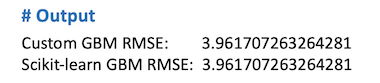As you can see in the output above, both models have exactly the same RMSE.

## Conclusion

The algorithm we have reviewed in this post is just one of the options of gradient boosting algorithm that is specific to regression problems with squared loss. You can find relevant code here and here.

Article originally posted here. Reposted with permission.## ODSC Community

The Open Data Science community is passionate and diverse, and we always welcome contributions from data science professionals! All of the articles under this profile are from our community, with individual authors mentioned in the text itself.

1